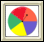Homework HelpMultiple-Choice Skills Practice Active Math Online Did You Know?Vocabulary Quiz

Statistics & Mean, Median, Mode

Graphing

• BBC Bitesize Graphing
• National Library of Virtual Manipulatives
•Bar Chart – Create a bar chart showing quantities or percentages by labeling columns and clicking on values.Histogram – Use this tool to summarize data using a histogram graph.Scatterplot – Plot multiple data points in two dimensions and determine correlation.Spinners – Work with spinners to learn about numbers and probabilities.
• Bar Graphs and Histograms ... what's the difference?
• Scatterplots and Lines of Best Fit ... learn how they work.
• Box & Whisker Plots ... how to make them, and what they mean.
• Shodor Interactives

Measurement

• Worsley School Precision
• AAAMath Measurement
• BBC Bitesize Measurement
• Factmonster Measurement
• National. Library of Virtual Manipulatives
•Converting Units – Use a simple system for converting units.Fill and Pour – Solve puzzles requiring you to fill and pour containers.

### Data About Us

Concept with Explanation
Selected Homework from ACE

The unit Data About Us was created to help students:

• Understand and use the process of data investigation by posing questions, collecting and analyzing data distributions, and making interpretations to answer questions;

• Represent data distributions using line plots, bar graphs, stem-and-leaf plots, and coordinate graphs;

• Compute the mean, median, or mode and the range of the data;

• Distinguish between categorical data and numerical data and identify which graphs and statistics may be used to represent each kind of data;

• Make informed decisions about which graph or graphs and which of the measures of center (mean, median, or mode) and range may be used to describe a data distribution; and

• Develop strategies for comparing data distributions.